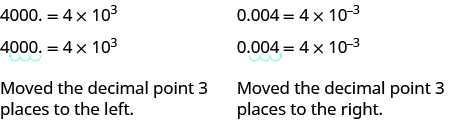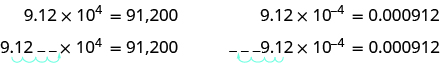## Scientific Notation

### Learning Outcomes

• Convert from decimal notation to scientific notation
• Convert from scientific notation to decimal notation
• Multiply and divide using scientific notation

### Convert from Decimal Notation to Scientific Notation

Remember working with place value for whole numbers and decimals? Our number system is based on powers of $10$. We use tens, hundreds, thousands, and so on. Our decimal numbers are also based on powers of tens—tenths, hundredths, thousandths, and so on.

Consider the numbers $4000$ and $0.004$. We know that $4000$ means $4\times 1000$ and $0.004$ means $4\times {\Large\frac{1}{1000}}$. If we write the $1000$ as a power of ten in exponential form, we can rewrite these numbers in this way:

$\begin{array}{cccc}4000\hfill & & & 0.004\hfill \\ 4\times 1000\hfill & & & 4\times {\Large\frac{1}{1000}}\hfill \\ 4\times {10}^{3}\hfill & & & 4\times {\Large\frac{1}{{10}^{3}}}\hfill \\ & & & \hfill 4\times {10}^{-3}\hfill \end{array}$

When a number is written as a product of two numbers, where the first factor is a number greater than or equal to one but less than $10$, and the second factor is a power of $10$ written in exponential form, it is said to be in scientific notation.

### Scientific Notation

A number is expressed in scientific notation when it is of the form

$a\times {10}^{n}$

where $a\ge 1$ and $a<10$ and $n$ is an integer.

It is customary in scientific notation to use $\times$ as the multiplication sign, even though we avoid using this sign elsewhere in algebra.

Scientific notation is a useful way of writing very large or very small numbers. It is used often in the sciences to make calculations easier.

If we look at what happened to the decimal point, we can see a method to easily convert from decimal notation to scientific notation.In both cases, the decimal was moved $3$ places to get the first factor, $4$, by itself.

• The power of $10$ is positive when the number is larger than $1\text{ : }4000=4\times {10}^{3}$.
• The power of $10$ is negative when the number is between $0$ and $1\text{ : }0.004=4\times {10}^{-3}$.

### example

Write $37,000$ in scientific notation.

Solution

 Step 1: Move the decimal point so that the first factor is greater than or equal to $1$ but less than $10$.Step 2: Count the number of decimal places, $n$ , that the decimal point was moved. $3.70000$ $4$ places Step 3: Write the number as a product with a power of $10$. $3.7\times {10}^{4}$ If the original number is: greater than 1, the power of 10 will be ${10}^{n}$ . between 0 and 1, the power of 10 will be ${10}^{\mathrm{-n}}$ Step 4: Check. ${10}^{4}$ is $10,000$ and $10,000$ times $3.7$ will be $37,000$. $37,000=3.7\times {10}^{4}$

### Convert from decimal notation to scientific notation

1. Move the decimal point so that the first factor is greater than or equal to $1$ but less than $10$.
2. Count the number of decimal places, $n$, that the decimal point was moved.
3. Write the number as a product with a power of $10$.
• If the original number is:
• greater than $1$, the power of $10$ will be ${10}^{n}$.
• between $0$ and $1$, the power of $10$ will be ${10}^{-n}$.
4. Check.

### example

Write in scientific notation: $0.0052$

### Convert Scientific Notation to Decimal Form

How can we convert from scientific notation to decimal form? Let’s look at two numbers written in scientific notation and see.

$\begin{array}{cccc}9.12\times {10}^{4}\hfill & & & 9.12\times {10}^{-4}\hfill \\ 9.12\times 10,000\hfill & & & 9.12\times 0.0001\hfill \\ 91,200\hfill & & & 0.000912\hfill \end{array}$

If we look at the location of the decimal point, we can see an easy method to convert a number from scientific notation to decimal form.In both cases the decimal point moved 4 places. When the exponent was positive, the decimal moved to the right. When the exponent was negative, the decimal point moved to the left.

### example

Convert to decimal form: $6.2\times {10}^{3}$

### Convert scientific notation to decimal form

1. Determine the exponent, $n$, on the factor $10$.
2. Move the decimal $n$ places, adding zeros if needed.
• If the exponent is positive, move the decimal point $n$ places to the right.
• If the exponent is negative, move the decimal point $|n|$ places to the left.
3. Check.

### example

Convert to decimal form: $8.9\times {10}^{-2}$

### Multiply and Divide Using Scientific Notation

We use the Properties of Exponents to multiply and divide numbers in scientific notation.

### example

Multiply. Write answers in decimal form: $\left(4\times {10}^{5}\right)\left(2\times {10}^{-7}\right)$.

### example

Divide. Write answers in decimal form: ${\Large\frac{9\times {10}^{3}}{3\times {10}^{-2}}}$.

### try it

The following video is a mini-lesson on how to convert decimals to scientific notation, and back to a decimal. Additionally, you will see more examples of how to multiply and divide numbers given in scientific notation.